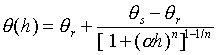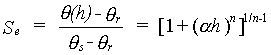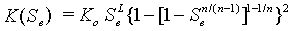ROSETTA Hydraulic FunctionsThe present version of Rosetta is capable of predicting van Genuchten (1980) water retention and unsaturated hydraulic conductivity parameters, as well as of providing estimates of the saturated hydraulic conductivity, Ks. The van Genuchten water retention function is given by:where ?(h) represents the water retention curve defining the water content, ? (cm3/cm3), as a function of the soil water pressure head h (cm), ?r and ?s (cm3/cm3) are residual and saturated water contents, respectively, while a (1/cm) and n are curve shape parameters. This equation can be rewritten to yield the relative saturation, Se:This equation is used in conjunction with the pore-size distribution model by Mualem (1976) to yield the van Genuchten-Mualem model (van Genuchten, 1980):n which Ko is the matching point at saturation (cm/day) and similar, but not necessarily equal, to the saturated hydraulic conductivity, Ks. The parameter L (-) is an empirical pore tortuosity/connectivity parameter that is normally assumed to be 0.5 (Mualem, 1976). Rosetta predicts L which will be negative in most cases. Although this leads to some theoretical complications, negative L values give far better results (cf., Kosugi, 1999; Schaap and Leij, 1999).